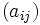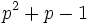# Difference between revisions of "Unitriangular matrix group:UT(3,p)"

View other such prime-parametrized groups

## Definition

### As a group of matrices

Given a prime$p$, the group$U(3,p)$ is defined as follows: it is the group of upper triangular matrices with 1s on the diagonal, and entries over$F_p$ (with the group operation being matrix multiplication).

Each such matrix$(a_{ij})$ can be described by the three entries$a_{12}, a_{13}, a_{23}$. The matrix looks like:$\begin{pmatrix} 1 & a_{12} & a_{13} \\ 0 & 1 & a_{23}\\ 0 & 0 & 1\end{pmatrix}$

The multiplication of matrices$A = (a_{ij})$ and$B = (b_{ij})$ gives the matrix$C = (c_{ij})$ where:

•$c_{12} = a_{12} + b_{12}$
•$c_{13} = a_{13} + b_{13} + a_{12}b_{23}$
•$c_{23} = a_{23} + b_{23}$

The analysis given below does not apply to the case$p = 2$. For$p = 2$, we get the dihedral group:D8, which is studied separately.

### As a semidirect product

This group of order$p^3$ can also be described as a semidirect product of the elementary abelian group of order$p^2$ by the cyclic group of order$p$, where the generator of the cyclic group of order$p$ acts via the automorphism:$(a,b) \mapsto (a,a+b)$

In this case, for instance, we can take the subgroup with$a_{12} = 0$ as the elementary abelian subgroup of order$p^2$ and the subgroup with$a_{23} = a_{13} = 0$ as the cyclic subgroup of order$p$.

## Families

1. These groups fall in the more general family$U(n,p)$ of unipotent upper-triangular matrices, which is the$p$-Sylow subgroup of the general linear group$GL(n,p)$. This further can be generalized to$U(n,q)$ where$q$ is the power of a prime$p$, which is the$p$-Sylow subgroup of$GL(n,q)$.
2. These groups also fall into the general family of extraspecial groups.

## Elements

### Upto conjugacy

Every element has order$p$.

The conjugacy classes are as follows:

1. The center has order precisely$p$, so there are$p$ elements that form conjugacy classes of size 1. These are, specifically, the elements with$a_{12} = a_{23} = 0$, and they're thus parametrized by their$a_{13}$ entry.
2. For every element outside the center, the centralizer of that element is the subgroup generated by that element and the center, and is hence of order$p^2$. Thus, the conjugacy class of the element is of size$p$. Thus, there is a total of$p^2 - 1$ conjugacy classes of size$p$.

### Upto automorphism

There are only three classes of elements upto automorphism:

1. The identity element, which forms a class of size 1
2. The non-identity elements in the center, which form a class of size$p - 1$
3. The non-central elements, which form a class of size$p^3 - p$

## Arithmetic functions

Compare and contrast arithmetic function values with other groups of prime-cube order at Groups of prime-cube order#Arithmetic functions

For some of these, the function values are different when$p = 2$ and/or when$p = 3$. These are clearly indicated below.

### Arithmetic functions taking values between 0 and 3

Function Value Explanation
prime-base logarithm of order 3 the order is$p^3$
prime-base logarithm of exponent 1 the exponent is$p^1$. Exception when$p = 2$, where the exponent is$2^2 = 4$.
nilpotency class 2
derived length 2
Frattini length 2
minimum size of generating set 2
subgroup rank 2
rank as p-group 2
normal rank as p-group 2
characteristic rank as p-group 1

### Arithmetic functions of a counting nature

Function Value Explanation
number of conjugacy classes$\! p^2 + p - 1$$p$ elements in the center, and each other conjugacy class has size$p$
number of subgroups$\! p^2 + 2p + 4$ when$p \ne 2$,$10$ when$p = 2$
number of normal subgroups$\! p + 4$
number of conjugacy classes of subgroups$\! 2p + 5$ for$p \ne 2$,$8$ for$p = 2$

## Subgroups

Further information: Subgroup structure of prime-cube order group:U(3,p) Here is the complete list of subgroups:

1. The trivial subgroup (1)
2. The center, which is a group of order$p$. In matrix terms, this is the subgroup comprising matrices$a_{ij}$ with$a_{12} = a_{23} = 0$. (1)
3. Subgroups of order$p$ generated by non-central elements. These are not normal, and occur in conjugacy classes of size$p$. ($p(p+1)$)
4. Subgroups of order$p^2$ containing the center. These are the inverse images via the quotient map by the center, of subgroups of order$p$ in the inner automorphism group. ($p + 1$)
5. The whole group. (1)

### Normal subgroups

The subgroups in (1), (2), (4) and (5) above are normal.

### Characteristic subgroups

The subgroups in (1), (2) and (5) above are normal. In other words, there are only three characteristic subgroups. Some notable facts:

## Subgroup-defining functions

Subgroup-defining function Subgroup type in list Isomorphism class Comment
Center (2) Group of prime order
Commutator subgroup (2) Group of prime order
Frattini subgroup (2) Group of prime order The$p + 1$ maximal subgroups of order$p^2$ intersect here.
Socle (2) Group of prime order This subgroup is the unique minimal normal subgroup, i.e.,the monolith, and the group is monolithic. Also, minimal normal implies central in nilpotent.

### Quotient-defining function

Quotient-defining function Isomorphism class Comment
Inner automorphism group Elementary abelian group of prime-square order It is the quotient by the center, which is of prime order.
Abelianization Elementary abelian group of prime-square order It is the quotient by the commutator subgroup, which is of prime order.
Frattini quotient Elementary abelian group of prime-square order It is the quotient by the Frattini subgroup, which is of prime order.

## GAP implementation

### GAP ID

For any prime$p$, this group is the third group among the groups of order$p^3$. Thus, for instance, if$p = 7$, the group is described using GAP's SmallGroup function as:

SmallGroup(343,3)

Note that we don't need to compute$p^3$; we can also write this as:

SmallGroup(7^3,3)

### As an extraspecial group

For any prime$p$, we can define this group using GAP's ExtraspecialGroup function as:

ExtraspecialGroup(p^3,'+')

For$p \ne 2$, it can also be constructed as:

ExtraspecialGroup(p^3,p)

where the argument$p$ indicates that it is the extraspecial group of exponent$p$. For instance, for$p = 5$:

ExtraspecialGroup(5^3,5)

## Endomorphisms

### Automorphisms

The automorphisms essentially permute the subgroups of order$p^2$ containing the center, while leaving the center itself unmoved.

## Related groups

For any prime$p$, there are (up to isomorphism) two non-abelian groups of order$p^3$. One of them is this, and the other is the semidirect product of the cyclic group of order$p^2$ by a group of order$p$ acting by power maps (with the generator corresponding to multiplication by$p+1$).